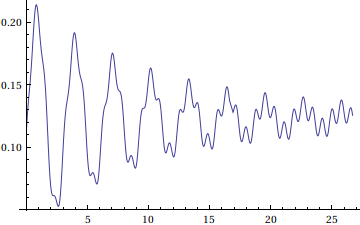# FESW: Equation solving in Mathematica

When:
January 1, 2014 @ 7:00 pm – 8:30 pm
2014-01-01T19:00:00-05:00
2014-01-01T20:30:00-05:00
Where:
Windows training rooms, 3rd floor of Duderstadt
Contact:
Jacob Davidson

Because of its vast capabilities for symbolic manipulation, Mathematica is unique in its ability to solve and analyze different types of equations. This workshops aims to introduce students to how to use Mathematica to analytically and numerically solve algebraic and differential equations. We will go over common example problems using Solve, FindRoot, DSolve, and NDSolve, such as a mass-spring-damper system in response to various forcing functions. We will also go over using Manipulate to dynamically change parameter values and view solution results.It will be assumed that students already have some basic familiarity with Mathematica. Some knowledge of differential equations will also be assumed. However, the focus will be on using Mathematica, and the relevant physics and mathematics will be explained before solving.

Please register using the form below